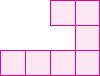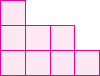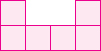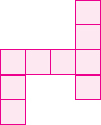# User ForumSubject :IMO    Class : Class 2

Which of the following has the maximum number of unit squares? (11 unit square)

ABCDAnswer is option (D), because the first figure has 7 squares.the second figure has 8 squares.the third figure has 6 squares and, the last figure has 9 squares. so the figure containing (more squares) squares is the last option that is (9) - option (D).... Thanks :D